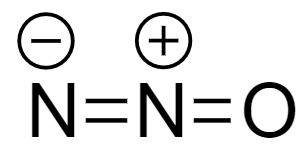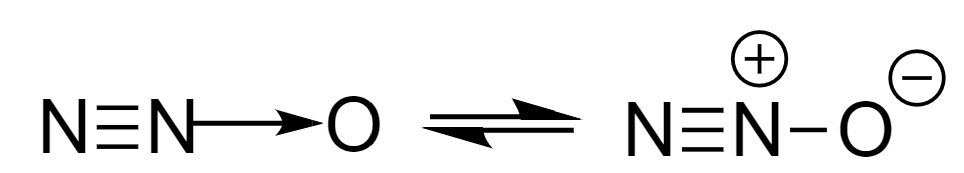Courses
Courses for Kids
Free study material
Free LIVE classes
More# Assertion: ${N_2}O$ is represented by $(i)\,N = N = O$ and $(ii)\,N \equiv N \to O$ but later is more stable.Reason: The form $(ii)$ shows resonance.(A) Both assertion and reason are correct and reason is the correct explanation for assertion.(B) Both assertion and reason are correct but reason is not the correct explanation for assertion.(C) Assertion is correct but reason is incorrect.(D) Assertion is incorrect but the reason is correct.

Last updated date: 18th Mar 2023
Total views: 204.6k
Views today: 1.84kVerified
204.6k+ views
Hint :Resonance: The delocalization of $\pi$ bond within the molecule or compound to form different canonical structures is known as resonance. It is the major factor due to which a molecule gains stability.

Nitrous oxide i.e., ${N_2}O$ is a non-flammable and colourless gas which is sweet in taste. It is also called laughing gas.
The two structures given for nitrous oxide are given as follows:
(i)(ii)As, the second structure is more stable than the first structure, so the assertion statement is correct.
The second structure is more stable than the first structure because of the arrangement of charges on the atoms on the basis of the electronegativity. In structure $(i)$ , the negative charge is on the nitrogen atom which is a less electronegative element whereas in structure $(ii)$ , the negative charge is on the oxygen atom which is the highly electronegative and a positive charge on the nitrogen atom which is comparatively less electronegative. Hence, the structure $(ii)$ forms a more stable canonical structure than $(i)$ and therefore more stable.
The reason statement given in the question is incorrect because both the structures of nitrous oxide are showing resonance. Here, the stability varies on the most stable canonical structure.
Hence, option (C) is the correct answer.

Note :
Canonical structures are also called resonance hybrids. The most stable canonical structures are those in which the most electronegative element is negatively charged and an atom which is comparatively less electronegative has a positive charge.